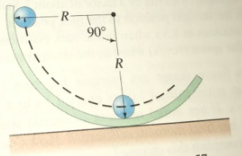# Problem: A ball of radius r rolls on the inside of a track of radius R. If the ball starts from rest at the vertical edge of the track, what will be its speed when it reaches the lowest point of the track, rolling without slipping?

###### FREE Expert Solution

From the conservation of energy,

The initial potential energy = Rotational energy.

$\begin{array}{rcl}\mathbf{m}\mathbf{g}\mathbf{\left(}\mathbf{R}\mathbf{-}\mathbf{r}\mathbf{\right)}& \mathbf{=}& \frac{\mathbf{1}}{\mathbf{2}}\mathbf{m}{\mathbf{v}}^{\mathbf{2}}\mathbf{+}\frac{\mathbf{1}}{\mathbf{2}}\mathbf{I}{\mathbf{\omega }}^{\mathbf{2}}\mathbf{+}\mathbf{P}\mathbf{.}{\mathbf{E}}_{\mathbf{f}}\end{array}$

But P.Ef = 0 since it is in motion.

and ω = v/r

93% (344 ratings)###### Problem Details

A ball of radius r rolls on the inside of a track of radius R. If the ball starts from rest at the vertical edge of the track, what will be its speed when it reaches the lowest point of the track, rolling without slipping?